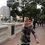# Problem Set 2

Hello! My intention for this week's problem set was a set about combinatorics. However, last week I couldn't study as much as I wanted, so I wasn't able to compile three nice enough problems in combinatorics. Sorry about that. Anyway, this set contains two problems in algebra (number 3 is particularly hard!!) and an easy geometry one. Please reshare the note if you liked it. Enjoy!

1. In a $\triangle{ABC}$, let $I$ be the incentre and let $X, Y, Z$ be the points of tangency of the incircle with sides $BC, AC, AB$, respectively. Line $AX$ cuts the incircle again in $P$, and line $AI$ cuts $YZ$ in $Q$. Prove that $X, I, Q, P$ lie on a circle. (Costa Rican OMCC TST 3, 2014)

2. Let $P(x)$ be a polynomial with integer coefficients such that there exist four distinct, positive integers $a, b, c, d$ which satisfy $P(a)=P(b)=P(c)=P(d)=5$. Show that there does not exist an integer $k$ which satisfies $P(k)=8$. (Canada, 1970)

3. Let $c$ be a positive integer. We define the sequence $x_n$ as follows: $x_1=c$, and for $n\geq{2}$, $x_n=x_{n-1}+\lfloor{\dfrac{2x_{n-1}-(n+2)}{n}}\rfloor$. Find a closed expression for $x_n$ in terms of $n$ and $c$. (Costa Rican OIM TST 1, 2014)Note by José Marín Guzmán
7 years ago

This discussion board is a place to discuss our Daily Challenges and the math and science related to those challenges. Explanations are more than just a solution — they should explain the steps and thinking strategies that you used to obtain the solution. Comments should further the discussion of math and science.

When posting on Brilliant:

• Use the emojis to react to an explanation, whether you're congratulating a job well done , or just really confused .
• Ask specific questions about the challenge or the steps in somebody's explanation. Well-posed questions can add a lot to the discussion, but posting "I don't understand!" doesn't help anyone.
• Try to contribute something new to the discussion, whether it is an extension, generalization or other idea related to the challenge.

MarkdownAppears as
*italics* or _italics_ italics
**bold** or __bold__ bold
- bulleted- list
• bulleted
• list
1. numbered2. list
1. numbered
2. list
Note: you must add a full line of space before and after lists for them to show up correctly
paragraph 1paragraph 2

paragraph 1

paragraph 2

[example link](https://brilliant.org)example link
> This is a quote
This is a quote
    # I indented these lines
# 4 spaces, and now they show
# up as a code block.

print "hello world"
# I indented these lines
# 4 spaces, and now they show
# up as a code block.

print "hello world"
MathAppears as
Remember to wrap math in $$ ... $$ or $ ... $ to ensure proper formatting.
2 \times 3 $2 \times 3$
2^{34} $2^{34}$
a_{i-1} $a_{i-1}$
\frac{2}{3} $\frac{2}{3}$
\sqrt{2} $\sqrt{2}$
\sum_{i=1}^3 $\sum_{i=1}^3$
\sin \theta $\sin \theta$
\boxed{123} $\boxed{123}$